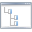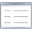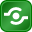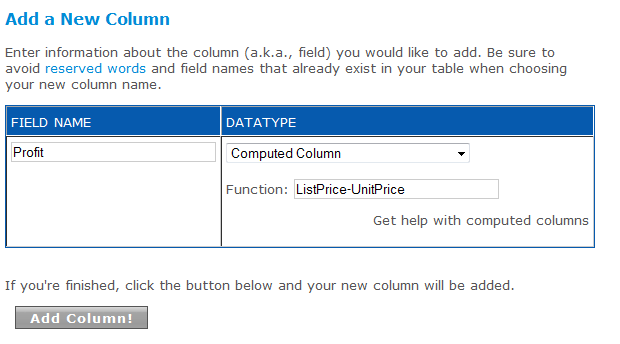TreeAlphabetical ListShare
Search:
id:
133

name:

Computed Column

description:

What is a Computed Column?

A Computed Column is a datatype that allows you to calculate mathematical equations using numerical data from other columns in your table, concatenate strings from other fields or add and subtract dates. For example, if you had a Products table with columns for price and tax rate, you could calculate price + tax. Computed columns allow you to take full advantage of Microsoft SQL Server 2005 Transact-SQL functions. You can read more about T-SQL functions at the MSDN Library.

You can only add a computed column after a table has been created. Go to your table, then go to Develop > Table Management. Enter a new column name under Add a column, and select computed column as the datatype.

Examples

Calculations with Numbers

Formulas with numbers are the same as we learned in school. To calculate Profit in a table with a Unit Price and a List Price, we enter a new column called Profit and in the formula box, enter "UnitPrice-ListPrice".Column with Dates

In this example, we calculate the number of days between two dates in our table. For more information about formulas you can use on dates, see the Date and Time Functions entry.Concatenating Fields

Concatenation is putting two strings together into one string. To add a concatenated field, use the + sign. To make a full name from a first and last name, enter a new column called FullName and in the formula box enter "Lastname + ', ' + Firstname". Click here to read more about T-SQL String Functions.

ParentTopic: